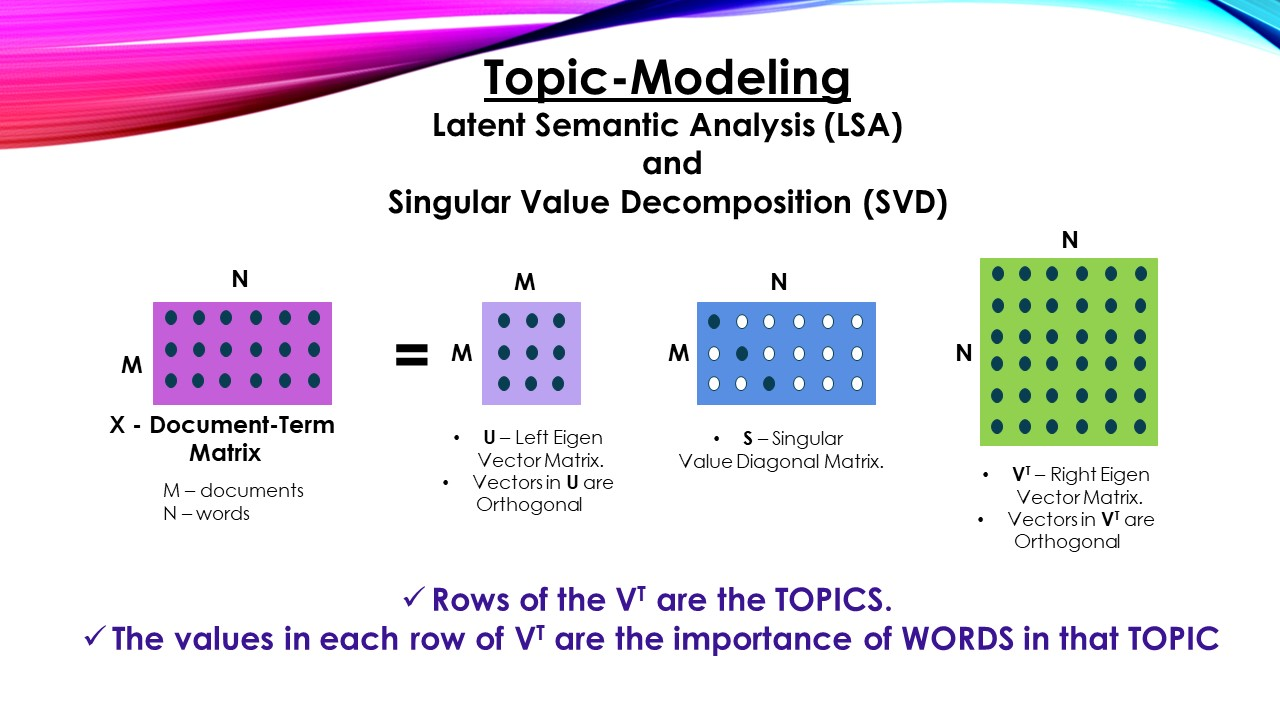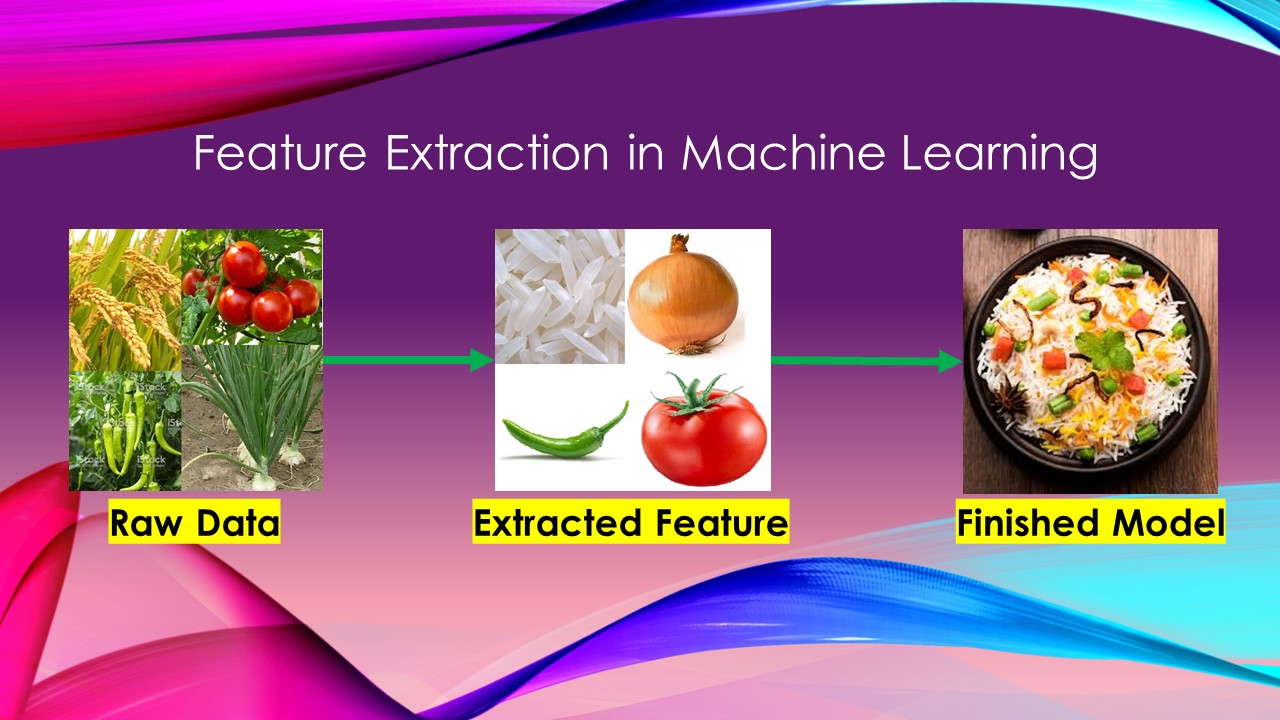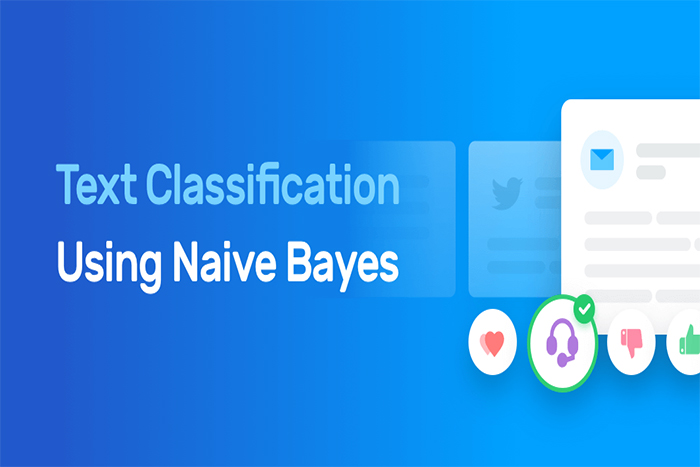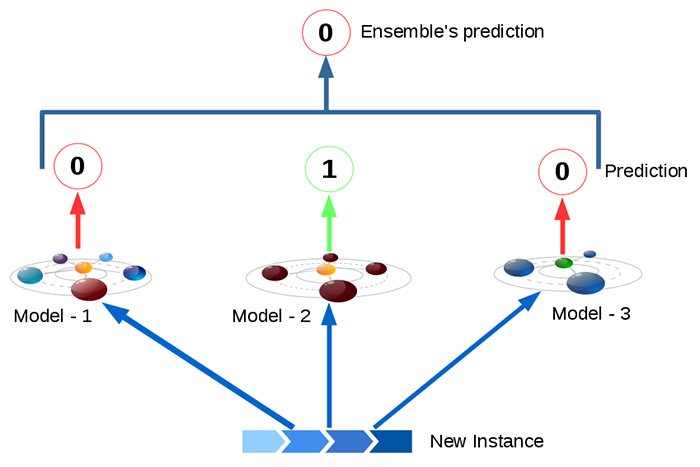Category: UncategorizedLatent Semantic Analysis (LSA) and Singular Value Decomposition (SVD)

Topic Modeling – Latent Semantic Analysis (LSA) and Singular Value Decomposition (SVD): Singular Value Decomposition is a Linear Algebraic concept used in may areas such as machine learning (principalFeature Engineering for Structured Data (numerical and categorical)

Feature Engineering for Structured Data (numerical and categorical) “Best Ingredients make Best Dish “, the same way “Best Features make Best Model” As part of any Machine Learning project,Regression – Find relation between Multiple Inputs and Target variable (Residuals Vs Fitted Graph)

Regression – Find relation between Multiple Inputs and Target variable One Input variable : When only one input variable and one output variable, scatter chart is useful in findingStep-by-Step Data Science Project (End to End Regression Model)

Step-by-Step Data Science Project (End to End Regression Model) We took “Melbourne housing market dataset from kaggle” and built a model to predict house price. While building the modelCredit Card Fraud Detection Using SMOTE

Credit Card Fraud Detection Using SMOTE (Classification approach) : This is the 2nd approach I’m sharing for credit card fraud detection. We are going to explore resampling techniques likeNaive Bayes Algorithm – Introduction to Text Analytics

Conditional Probability¶ Conditional probability as the name suggests, comes into play when the probability of occurrence of a particular event changes when one or more conditions are satisfied (these